### Home > CALC > Chapter 8 > Lesson 8.3.3 > Problem8-125

8-125.
1. Given: f(x) =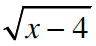Homework Help ✎

1. Find the area of the region bounded by f, x-axis, and the line x = 8.

2. Find the line x = c that will divide the region from part (a) into two equal pieces.

3. Find the volume of the solid that is formed by rotating the region described in part (a) about the x-axis.

4. Find a value d such that a plane perpendicular to the x-axis at d = x will divide the solid in part (c) into two equal parts.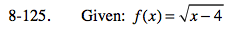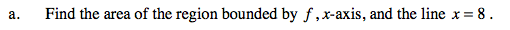Before you setup an integral, sketch the graph and shade the region. You should be able to sketch f(x) without a graphing calculator, as it is simply of horizontal shift of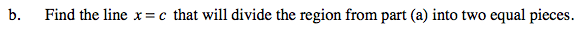Solve for c.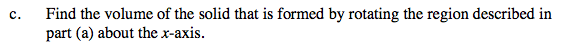Use disks.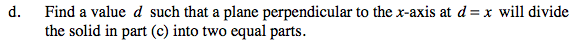Refer to the hint in part (b) and follow a similar process using a generic volume formula instead of a generic area formula.

$\text{Solve:}\int_4^d \pi(\sqrt{x-4})^2dx=\int_d^8\pi(\sqrt{x-4})^2dx$

$\frac{d^2}{2}-4d+8=-\frac{d^2}{2}+4d$